# Orthogonal transformation

(diff) ← Older revision | Latest revision (diff) | Newer revision → (diff)

A linear transformationof a Euclidean space preserving the lengths (or, equivalently, the scalar product) of vectors. Orthogonal transformations and only they can transfer an orthonormal basis to an orthonormal one. The equality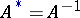is also a necessary and sufficient condition of orthogonality, whereis the conjugate and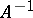the inverse linear transformation.

With respect to an orthonormal basis, orthogonal matrices correspond to orthogonal transformations and only to them. The eigen values of an orthogonal transformation are equal to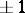, while the eigen vectors which correspond to different eigen values are orthogonal. The determinant of an orthogonal transformation is equal to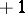(special orthogonal transformation) or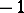(non-special orthogonal transformation). In the Euclidean plane, every special orthogonal transformation is a rotation, and its matrix in an appropriate orthonormal basis has the form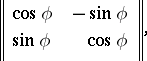whereis the angle of the rotation; and every non-special orthogonal transformation is a reflection with respect to a straight line through the origin, and its matrix in an appropriate orthonormal basis has the formIn three-dimensional space, every special orthogonal transformation is a rotation around an axis, while every non-special orthogonal transformation is the product of such a rotation and a reflection in a perpendicular plane. In an arbitrary-dimensional Euclidean space, orthogonal transformations also reduce to rotations and reflections (see Rotation).

The set of all orthogonal transformations in a Euclidean space is a group with respect to multiplication of transformations — the orthogonal group of the given Euclidean space. The special orthogonal transformations form a normal subgroup in this group (the special orthogonal group).